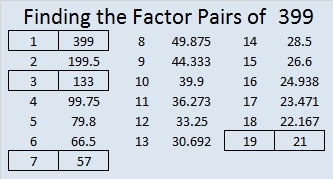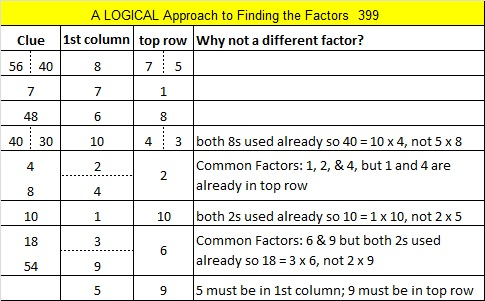# 399 and Level 5

399 is 400 – 1. Since 20 x 20 = 400, it is VERY easy to know one of the factor pairs for 399. It will be 399 = (20 – 1) x (20 + 1).Print the puzzles or type the factors on this excel file: 10 Factors 2015-02-16

• 399 is a composite number.
• Prime factorization: 399 = 3 x 7 x 19
• The exponents in the prime factorization are 1, 1, and 1. Adding one to each and multiplying we get (1 + 1)(1 + 1)(1 + 1) = 2 x 2 x 2 = 8. Therefore 399 has exactly 8 factors.
• Factors of 399: 1, 3, 7, 19, 21, 57, 133, 399
• Factor pairs: 399 = 1 x 399, 3 x 133, 7 x 57, or 19 x 21
• 399 has no square factors that allow its square root to be simplified. √399 ≈ 19.975This site uses Akismet to reduce spam. Learn how your comment data is processed.